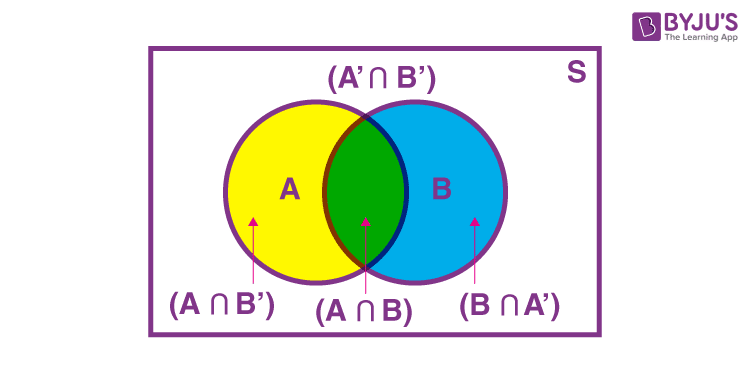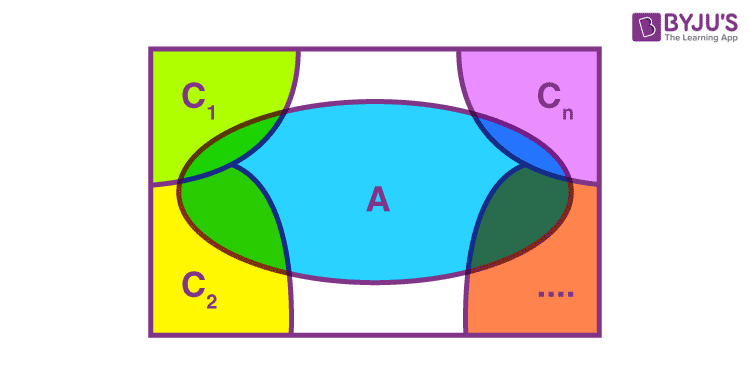# Total Probability Theorem

In Mathematics, probability is the likelihood of an event. The probability of an event going to happen is 1 and for an impossible event is 0. In probability theory, there exists a  fundamental rule that relates to the marginal probability and the conditional probability, which is called the formula or the law of total probability. Through several distinct events, it expresses the total probability of an event. Here, we are going to discuss the law of total probability, its statement, proof along with its example.

## Law of Total Probability

For two events A and B associated with a sample space S, the sample space can be divided into a set A ∩ B′, A ∩ B, A′ ∩ B, A′ ∩ B′. This set is said to be mutually disjoint or pairwise disjoint because any pair of sets in it is disjoint. Elements of this set are better known as a partition of sample space.

This can be represented by the Venn diagram as shown below. In cases where the probability of occurrence of one event depends on the occurrence of other events, we use the law of total probability theorem.## Law of Total Probability Statement

Let events C1, C2 . . . Cn form partitions of the sample space S, where all the events have a non-zero probability of occurrence. For any event, A associated with S, according to the total probability theorem,

$$\begin{array}{l} P(A) =\sum\limits_{k=0}^{n} P(C_k)P(A| C_k)\end{array}$$## Total Probability Theorem Proof

From the above-mentioned figure, {C1, C2, . . . . , Cn} is the partitions of the sample space S such that, Ci ∩ Ck = φ, where i ≠ k and i, k = 1, 2,…,n also all the events C1, C2 . . . . Cn have non zero probability. Sample space S can be given as,

S = C1 ∪ C2 ∪ . . . . . ∪ Cn

For any event A,

A = A ∩ S

= A ∩ (C1 ∪ C2∪ . . . . ∪ Cn)

= (A ∩ C1) ∪ (A ∩ C2) ∪ … ∪ (A ∩ Cn) . . . . . (1)

We know that A ∩ Ci and A ∩ Ck are the subsets of Ci and Ck. Here, Ci and Ck are disjoint for i ≠ k. since they are mutually independent events which implies that A ∩ Ci and A ∩ Ck are also disjoint for all i ≠ k. Thus,

P(A) = P [(A ∩ C1) ∪ (A ∩ C2) ∪ ….. ∪ (A ∩ Cn)]

= P (A ∩ C1) + P (A ∩ C2) + … + P (A ∩ Cn) . . . . . . . (2)

We know that,

P(A ∩ Ci) = P(Ci) P(A|Ci)(By multiplication rule of probability) . . . . (3)

Using (2) and (3), (1) can be rewritten as,

P(A) = P(C1)P(A| C1) + P(C2)P(A|C2) + P(C3)P(A| C3) + . . . . . + P(Cn)P(A| Cn)

Hence, the theorem can be stated in form of equation as,

$$\begin{array}{l}P(A) = \sum\limits_{k=0}^{n} P(C_k)P(A| C_k)\end{array}$$

### Total Probability Theorem Example

Example:

A person has undertaken a mining job. The probabilities of completion of the job on time with and without rain are 0.42 and 0.90 respectively. If the probability that it will rain is 0.45, then determine the probability that the mining job will be completed on time.

Solution:

Let A be the event that the mining job will be completed on time and B be the event that it rains. We have,

P(B) = 0.45,

P(no rain) = P(B′) = 1 − P(B) = 1 − 0.45 = 0.55

By multiplication law of probability,

P(A|B) = 0.42

P(A|B′) = 0.90

Since, events B and B′ form partitions of the sample space S, by total probability theorem, we have

P(A) = P(B) P(A|B) + P(B′) P(A|B′)

=0.45 × 0.42 + 0.55 × 0.9

= 0.189 + 0.495 = 0.684

So, the probability that the job will be completed on time is 0.684.

For a detailed discussion on the concept of the law of total probability theorem, download BYJU’S-The learning app.

Quiz on Total Probability Theorem